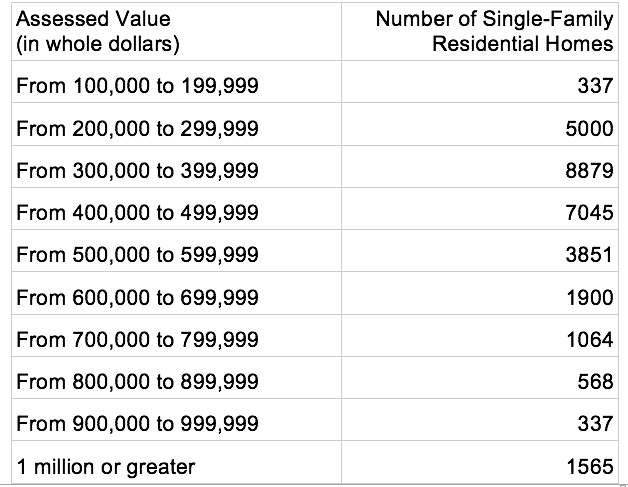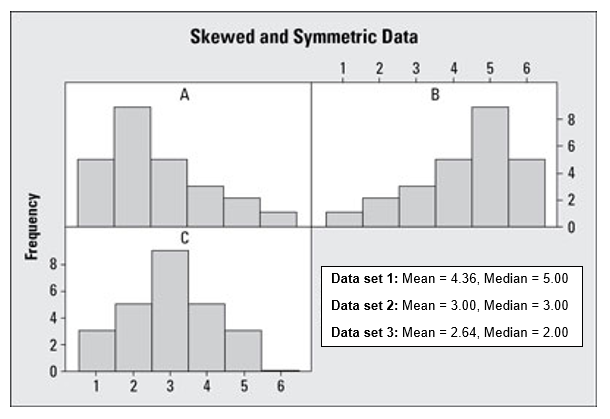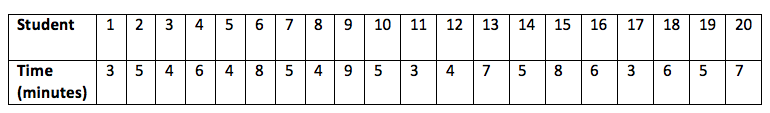# Descriptive Statistics

## Objective

Represent data in a histogram and calculate the center. Identify when the median and mean are not the same value.

## Common Core Standards

### Core Standards

?

• HSS-ID.A.1 — Represent data with plots on the real number line (dot plots, histograms, and box plots).

?

• 6.SP.B.4

• 7.SP.B.4

## Criteria for Success

?

1. Understand that histograms are a way to organize univariate data and identify the variable that the histogram is describing.
2. Create histograms using appropriate bin width and scale.
3. Find the mean and median of a data set, and mark these in the histogram.
4. Describe why the mean and median may not be in the same location. Make conjectures about the data set based on the location of the mean and the median.

## Tips for Teachers

?

In this lesson, students are asked to observe some differences between mean and median in a data set but will not need to be proficient at relating this to the skew of the data set until the end of lesson 4.

## Anchor Problems

?

### Problem 1

The information in the table represents the number of single-family residential homes in Boston and their respective assessed value for fiscal year 2017.

Sketch a histogram based on the data in the table.#### References

Analyze Boston Property Assessment

Property Assessment published by Department of Innovation and Technology is made available by Analyze Boston under the Open Data Commons PDDL. Accessed June 26, 2017, 2:31 p.m..

### Problem 2

Identify which histograms belong with the measures of center shown. Explain your reasoning.## Problem Set

?

The following resources include problems and activities aligned to the objective of the lesson that can be used to create your own problem set.

• Use as many real data sets as possible for the problems. Follow the set of guidelines for technology to also give students several different entry points for creating a histogram. It isn’t necessary for them to always start with a raw data set.
• Give students error analysis problems where the bins overlap or are missing values, and ask students to determine what is wrong.
• Find the bin with the mean and the median in it and predict the shape of the histogram based on this. (This will be formalized in the next lesson).
• Give students already-created histograms to analyze. Compare the representation in a histogram with that of a box plot.